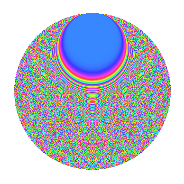# Properties

 Label 525.4.bvLevel $525$ Weight $4$ Character orbit 525.bv Rep. character $\chi_{525}(52,\cdot)$ Character field $\Q(\zeta_{60})$ Dimension $1920$ Sturm bound $320$

# Related objects

## Defining parameters

 Level: $$N$$ $$=$$ $$525 = 3 \cdot 5^{2} \cdot 7$$ Weight: $$k$$ $$=$$ $$4$$ Character orbit: $$[\chi]$$ $$=$$ 525.bv (of order $$60$$ and degree $$16$$) Character conductor: $$\operatorname{cond}(\chi)$$ $$=$$ $$175$$ Character field: $$\Q(\zeta_{60})$$ Sturm bound: $$320$$

## Dimensions

The following table gives the dimensions of various subspaces of $$M_{4}(525, [\chi])$$.

Total New Old
Modular forms 3904 1920 1984
Cusp forms 3776 1920 1856
Eisenstein series 128 0 128

## Trace form

 $$1920q - 48q^{5} + 4q^{7} - 168q^{8} + O(q^{10})$$ $$1920q - 48q^{5} + 4q^{7} - 168q^{8} + 72q^{10} + 168q^{15} - 3840q^{16} + 816q^{22} - 816q^{23} - 152q^{25} + 92q^{28} + 320q^{29} + 192q^{30} + 672q^{32} + 108q^{33} - 104q^{35} + 17280q^{36} + 552q^{37} - 10896q^{38} - 2052q^{40} + 1068q^{42} - 1840q^{43} - 240q^{47} - 14112q^{50} + 18576q^{52} + 4192q^{53} - 1392q^{57} - 512q^{58} - 9840q^{59} - 420q^{60} + 288q^{63} + 19320q^{64} + 824q^{65} + 3784q^{67} + 8844q^{68} - 2064q^{70} + 756q^{72} + 3312q^{73} + 1872q^{75} + 6600q^{77} + 1200q^{78} - 12108q^{80} - 19440q^{81} - 1764q^{82} - 22560q^{84} - 4768q^{85} - 5652q^{87} - 884q^{88} + 2152q^{92} + 5472q^{93} - 4768q^{95} + 34136q^{98} + O(q^{100})$$

## Decomposition of $$S_{4}^{\mathrm{new}}(525, [\chi])$$ into newform subspaces

The newforms in this space have not yet been added to the LMFDB.

## Decomposition of $$S_{4}^{\mathrm{old}}(525, [\chi])$$ into lower level spaces

$$S_{4}^{\mathrm{old}}(525, [\chi]) \cong$$ $$S_{4}^{\mathrm{new}}(175, [\chi])$$$$^{\oplus 2}$$

## Hecke characteristic polynomials

There are no characteristic polynomials of Hecke operators in the database